# Power and measuring itPage 4

#### WATCH ALL SLIDES

23

RGUIIIT BASAR

Slide 24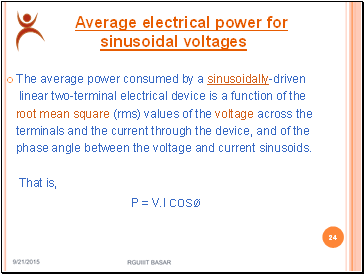## Average electrical power for sinusoidal voltages

The average power consumed by a sinusoidally-driven

linear two-terminal electrical device is a function of the

root mean square (rms) values of the voltage across the

terminals and the current through the device, and of the

phase angle between the voltage and current sinusoids.

That is,

P = V.I cosø

9/3/2015

24

RGUIIIT BASAR

Slide 25## Average electrical power for sinusoidal voltages

where

P is the average power, measured in watts

I is the root mean square value of the sinusoidal

alternating current (AC), measured in amperes

V is the root mean square value of the sinusoidal

alternating voltage, measured in volts

φ is the phase angle between the voltage and the current sine functions.

9/3/2015

25

RGUIIIT BASAR

Contd

Slide 26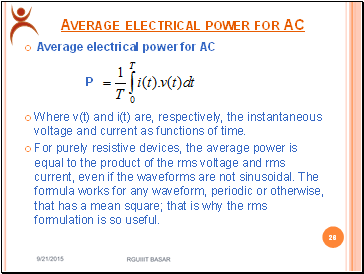## Average electrical power for AC

Average electrical power for AC

P

Where v(t) and i(t) are, respectively, the instantaneous voltage and current as functions of time.

For purely resistive devices, the average power is equal to the product of the rms voltage and rms current, even if the waveforms are not sinusoidal. The formula works for any waveform, periodic or otherwise, that has a mean square; that is why the rms formulation is so useful.

9/3/2015

26

RGUIIIT BASAR

Slide 27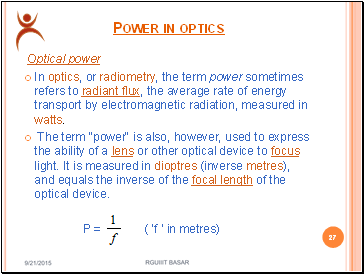## Power in optics

Optical power

In optics, or radiometry, the term power sometimes refers to radiant flux, the average rate of energy transport by electromagnetic radiation, measured in watts.

The term "power" is also, however, used to express the ability of a lens or other optical device to focus light. It is measured in dioptres (inverse metres), and equals the inverse of the focal length of the optical device.

P = ( ‘f ‘ in metres)

9/3/2015

27

RGUIIIT BASAR

Slide 28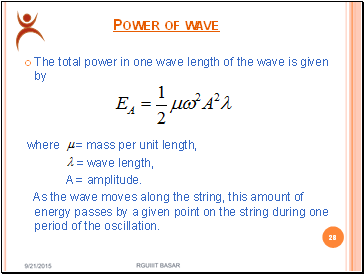## Power of wave

The total power in one wave length of the wave is given by

where = mass per unit length,

= wave length,

A = amplitude.

As the wave moves along the string, this amount of energy passes by a given point on the string during one period of the oscillation.

9/3/2015

28

RGUIIIT BASAR

Slide 29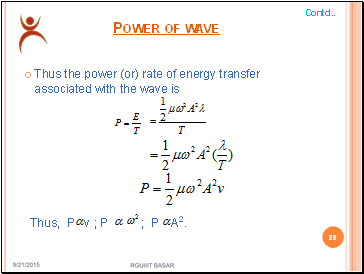Power of wave

Thus the power (or) rate of energy transfer associated with the wave is

Thus, P v ; P ; P A2.

Go to page:
1  2  3  4  5